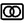# Power analyzerChristoph Tack wrote 10/20/2019 at 09:26 • 3 min read • Like

One of the applications of a differential voltage probe is to measure voltage over a sense resistor.  For low power applications, the current drawn can be quite small, which leads us to using a high value sense resistor.  Unfortunately this also increases the source resistance of our voltage supply.  So when the current suddenly rises (e.g. because the device wakes up), this might lead to a sharp drop in supply voltage to the device, even powering it down in the worst case.
The Elektor MicroSupply (Elektorlabs 9/10 2019) is Elektor's solution to measure power of low power devices.  It has the following features:

• built in ADC to measure voltage & current : we will use an oscilloscope for that, so that we're able to measure fast dynamic behavior (>1kHz sampling).
• built in DAC to set power supply voltage : we will use an analog input pin.  The voltage that the user connects to that pin will be used as output voltage.  The user can simply connect the 3V3, 5V or another voltage that is already present in their device.  No need for a DAC.
• The power supply itself also has its issues.  An opamp is used as active element in the control loop.  The supply voltage is limited by the opamp supply voltage.  The opamp itself is quite expensive >\$2.
• Elektor tackled the problem of the big dynamic range by using a 100ohm sense resistor and using three LT1789 instrumentation amplifiers, each set to a different amplification factor : 1x, 10x & 100x.  This has some downsides:
• Two types of LT1789 are needed.
• The instrumentation amplifiers are expensive : \$3.70/pce.
• The VoutMin of the LT1789 is 100mV.  So an extra offset is required.  This is provided by an LT1790, another expensive part from Linear.
• A BC847 is used as pass transistor.  This seems a bit under powered:
• VinMax = 12V
• VoutMin = 1.5V
• IoutMax = 40mA
• => P(T1) = (12-1.5)*0.040 = 420mW, which is too much for the 250mW BC847.

The Elektor designer also thought of using a single instrumentation amplifier with switchable sense resistors, but they didn't see the benefit from it.

# Cheaper implementation

Single differential amplifiers with multiple sense resistorsHigh side potentiostat to overcome the burden voltage (=voltage drop over the sense resistor).

The working principle of this power supply is quite simple.  Some calculations:

• V(R4) = V1 - Vka(U2) = 3.3 - 1.24V
• Vout = V(R4) + Vref(U1) = (3.3 -1.24) + 1.24 = 3.3V

So the power supply output voltage is equal to the analog input voltage V1.

The sense resistor R2 is switchable with the gate of M1.  There could be many PMOS-transistor / sense resistor combinations mounted in parallel with M1 & R2.

# Low current measuring techniques

Many sources refer to the transimpedance amplifier.  The advantage is that it avoids big sense resistors and big burden voltages.  The disadvantage is that a negative power supply rail is needed.

# Commercial solutions

## Discussions